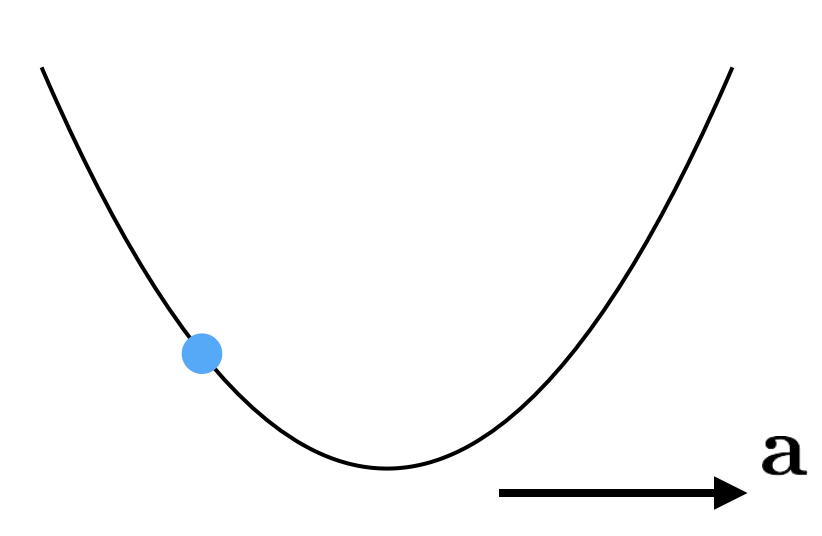# Extremum Accelerometer

A smooth wire is bent into the shape of a parabola. If the origin is taken to be the lowest point on the wire, $x$ and $y$ are related by $y=x^2$. The wire starts accelerating to the right with acceleration $\mathbf{a}$. Suppose the value of $x_\textrm{ss}$, the steady state location of the bead in the coordinates of the wire, is $-\SI{30}{\centi\meter}$.Find the acceleration $\mathbf{a}$ (in $\si[per-mode=symbol]{\meter\per\second\squared}$) of the wire toward the right.

Details and Assumptions:

• $g = \SI[per-mode=symbol]{10}{\meter\per\second\squared}$.
×

Problem Loading...

Note Loading...

Set Loading...